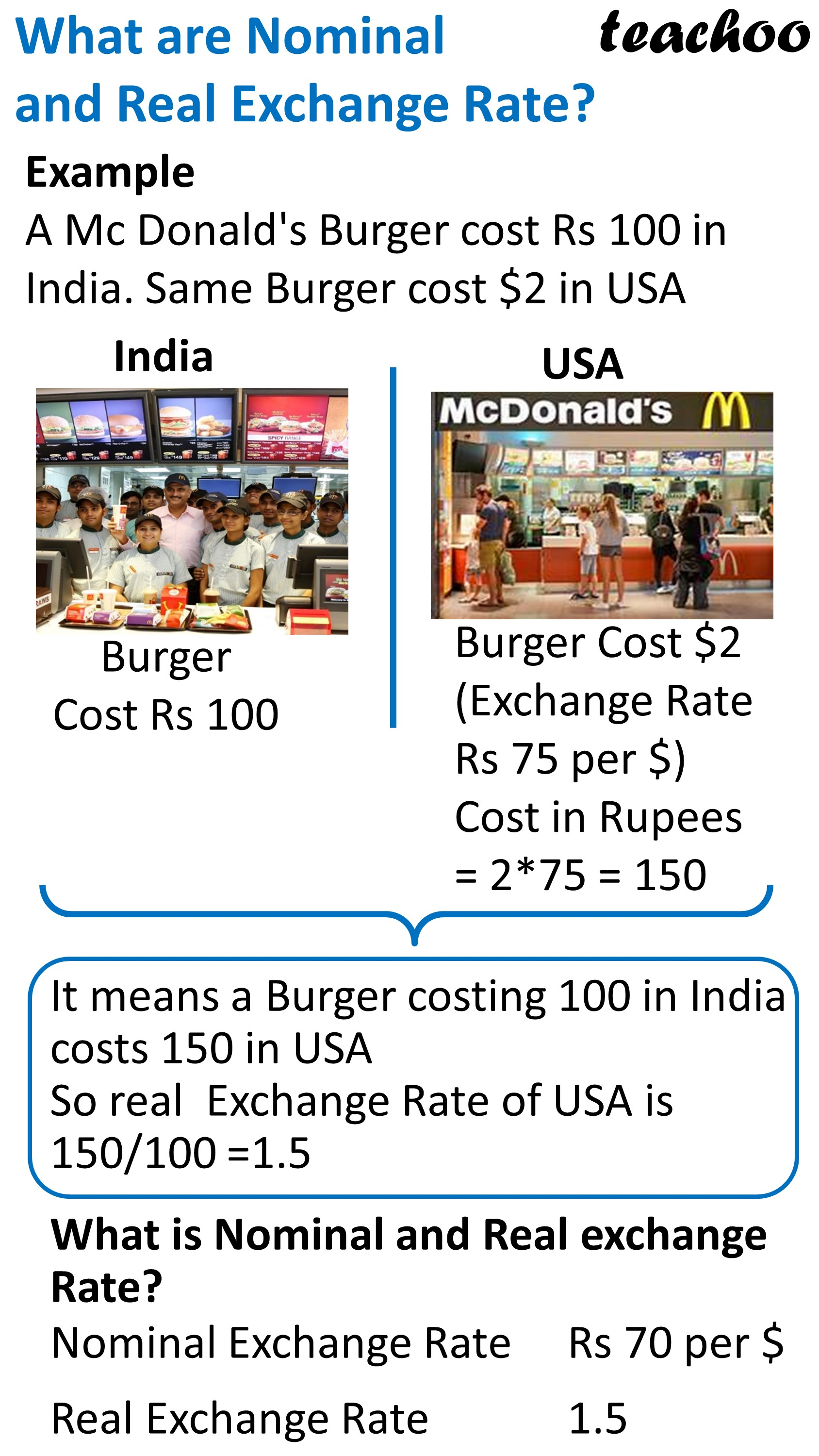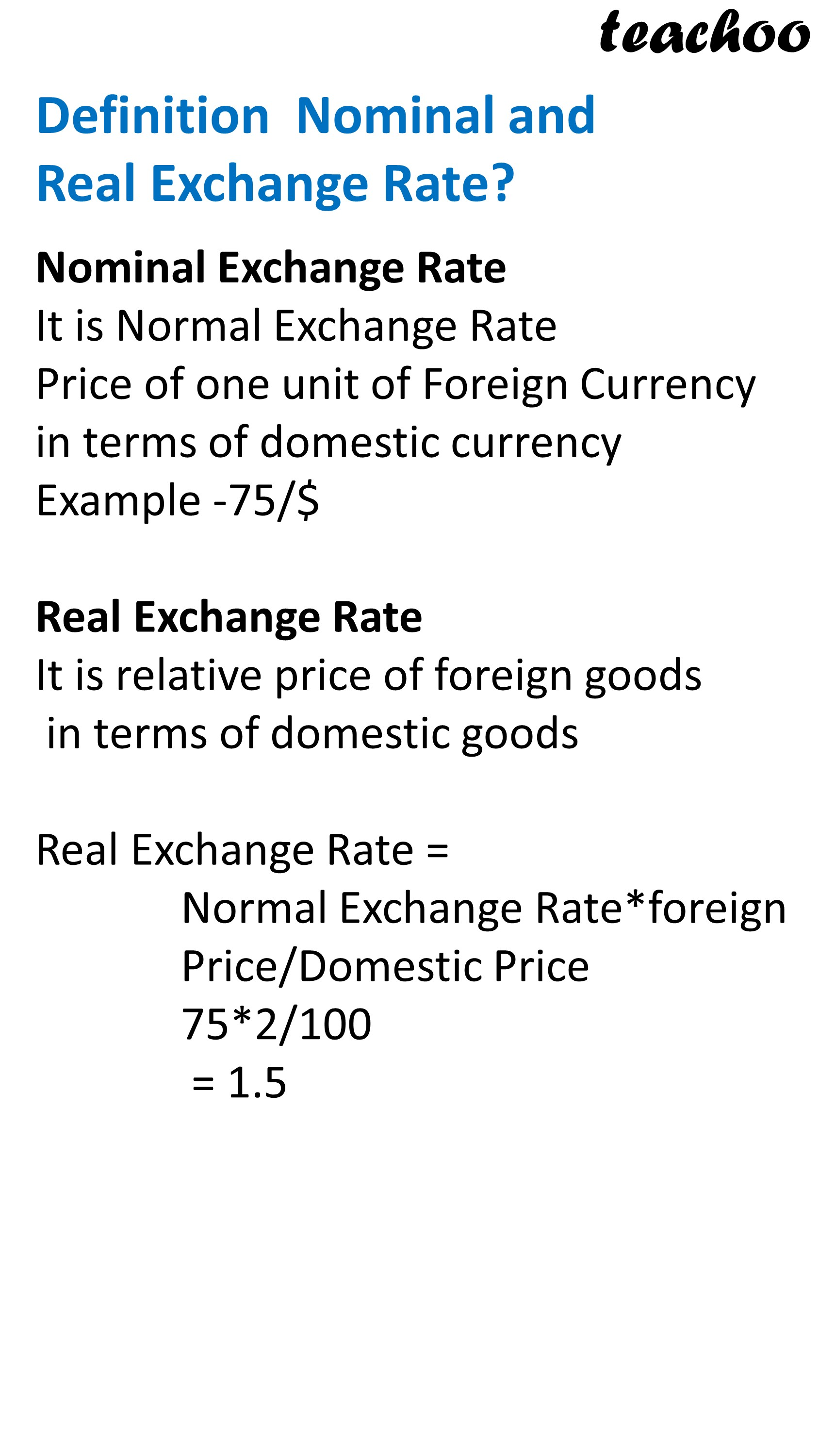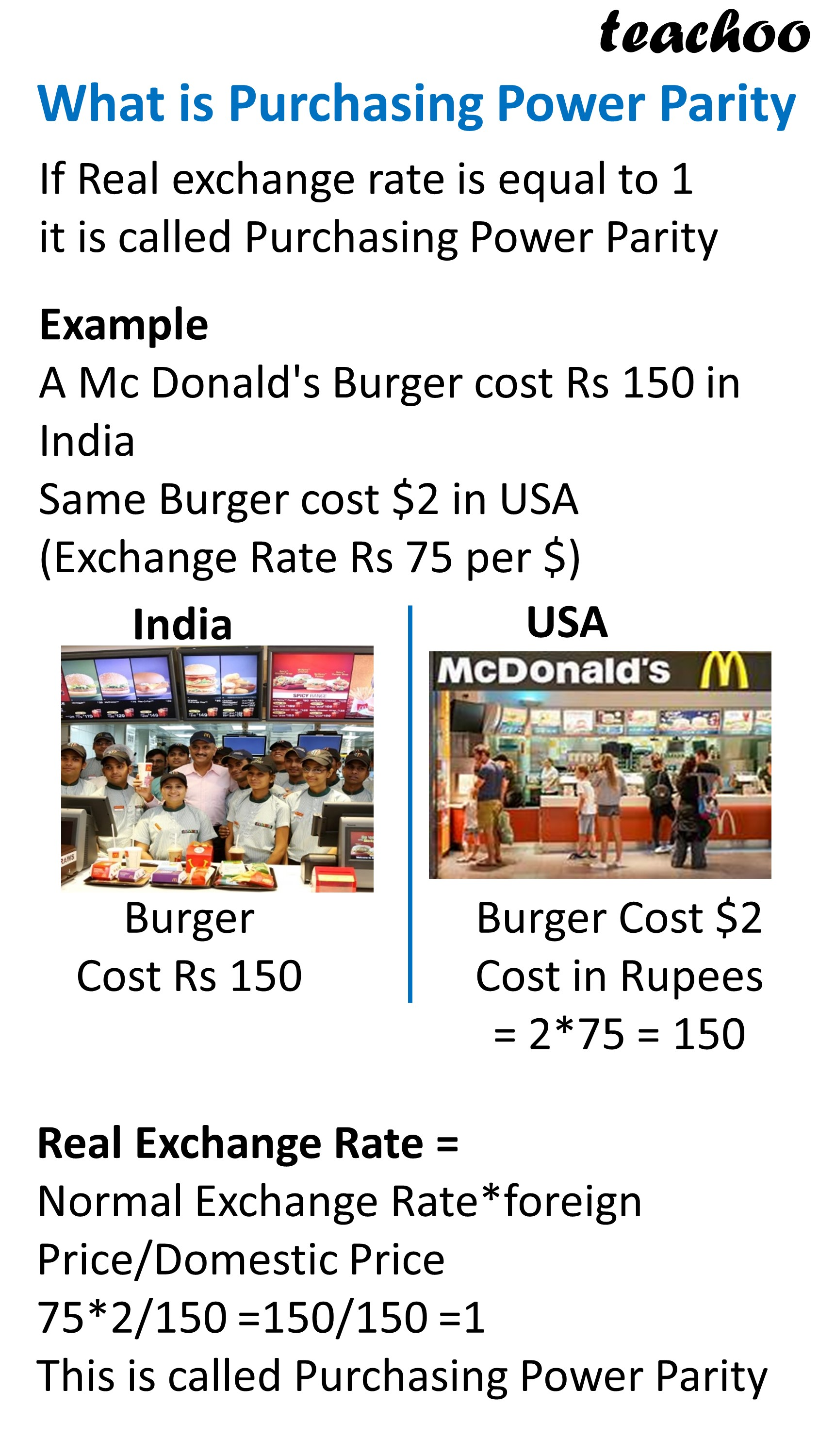Chapter 6 Part 1 - Foreign Exchange Rates

Economics Class 12
Macroeconomics

## What is Nominal Exchange Rate?

The nominal exchange rate is the price of one unit of foreign currency in terms of domestic currency

Example

Dollar Rate is 75/Dollar

It means one unit of foreign currency dollar is equal to 75 Rupees

## What is Real Exchange Rate?

It is relative price of foreign goods in terms of domestic goods

Real Exchange Rate = Normal Exchange Rate * Foreign Price/ Domestic Price## What is Purchasing Power Parity?

When Real Exchange Rate is equal to 1

it is called Purchasing Power parity

Example

Suppose a burger cost Rs 140 in India and 2 Dollar in USA

Exchange Rate is Rs 70/Dollar

Real Exchange Rate = Normal Exchange Rate * Foreign Price/ Domestic Price

= 70*2/140

= 140/140

= 1

### NCERT Questions

#### Question 3

Distinguish between the nominal exchange rate and the real exchange rate.

If you were to decide whether to buy domestic goods or foreign goods, which rate would be more relevant?

Explain.

### Other Books

#### Question 1

In the following questions, select the correct answers:

How is Real exchange rate calculated?

1. Normal Exchange Rate * Domestic Price/ Foreign Price
2. Normal Exchange Rate * Foreign Price/ Foreign Price
3. Normal Exchange Rate * Foreign Price/ Domestic Price
4. Real Exchange Rate * Foreign Price/ Domestic Price

#### Question 2

1. When Real Exchange Rate is less than Nominal Exchange Rate it is called Purchasing Power parity.
2. When Real Exchange Rate is equal to 1 it is called Purchasing Power parity.
3. When Real Exchange Rate is greater than Nominal Exchange Rate it is called Purchasing Power parity.
4. None of the above

Learn in your speed, with individual attention - Teachoo Maths 1-on-1 Class

### Transcript

What are Nominal and Real Exchange Rate? Example A Mc Donald's Burger cost Rs 100 in India. Same Burger cost \$2 in USA India USA Burger Cost Rs 100 Burger Cost \$2 (Exchange Rate Rs 75 per \$) Cost in Rupees = 2*75 = 150 It means a Burger costing 100 in India costs 150 in USA So real Exchange Rate of USA is 150/100 =1.5 What is Nominal and Real exchange Rate? It means a Burger costing 100 in India costs 150 in USA So real Exchange Rate of USA is 150/100 =1.5 What is Nominal and Real exchange Rate? Nominal Exchange Rate Real Exchange Rate Definition Nominal and Real Exchange Rate? Nominal Exchange Rate It is Normal Exchange Rate Price of one unit of Foreign Currency in terms of domestic currency Example -75/\$ Real Exchange Rate It is relative price of foreign goods in terms of domestic goods Real Exchange Rate = Normal Exchange Rate*foreign Price/Domestic Price 75*2/100 = 1.5 What is Purchasing Power Parity If Real exchange rate is equal to 1 it is called Purchasing Power Parity Example A Mc Donald's Burger cost Rs 150 in India Same Burger cost \$2 in USA (Exchange Rate Rs 75 per \$) Burger Cost Rs 150 Burger Cost \$2 Cost in Rupees = 2*75 = 150 Real Exchange Rate = Normal Exchange Rate*foreign Price/Domestic Price 75*2/150 =150/150 =1 This is called Purchasing Power Parity Example 2 – Purchasing Power Parity A Mc Donald's Burger cost Rs 100 in India Same Burger cost \$2 in USA (Exchange Rate Rs 50 per \$) Burger Cost Rs 100 Burger Cost \$2 Cost in Rupees = 2*50 = 100 Real Exchange Rate = Normal Exchange Rate*foreign Price/Domestic Price 50*2/100 =100/100 =1 This is called Purchasing Power Parity